### Home > CAAC > Chapter 1 > Lesson 1.2.1 > Problem1-49

1-49.
1. Try these problems Without a calculator first. Then use a calculator to check your answer. Homework Help ✎

1. −16 + 7

2. 10 − (−24)

3. (3)(−9)

4. −9 + (−11)

5. −49 − 36

6. −56 ÷ (−7)

7. 15 ÷ (−3)

8. −7 ÷ 7

9. (−6) · 9

10. (−5)(−5)

11. (−6) · (43)

12. 27 − (−3) − 4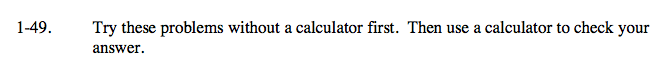Remember: When you subtract a negative, it changes to adding a positive. When you multiply two negatives, they become positive.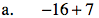−9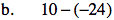34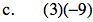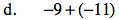−20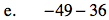−85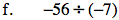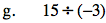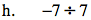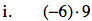−54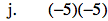25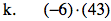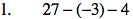26